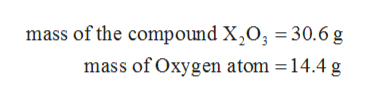# A 30.6 g sample of the compound X2O3 contains 14.4 g of oxygen atoms. What is the molar mass of element X?

Question
89 views

A 30.6 g sample of the compound X2O3 contains 14.4 g of oxygen atoms. What is the molar mass of element X?

check_circle

Step 1

The values are given as follows:help_outlineImage Transcriptionclosemass of the compound X203 30.6 g mass of Oxygen atom = 14.4 g fullscreen
Step 2

The number of moles of oxygen atom can be calculated as follows:

Step 3

Since, the mole ratio of X and O is 2:3. Therefore, the numbe...

### Want to see the full answer?

See Solution

#### Want to see this answer and more?

Solutions are written by subject experts who are available 24/7. Questions are typically answered within 1 hour.*

See Solution
*Response times may vary by subject and question.
Tagged in

### Chemistry